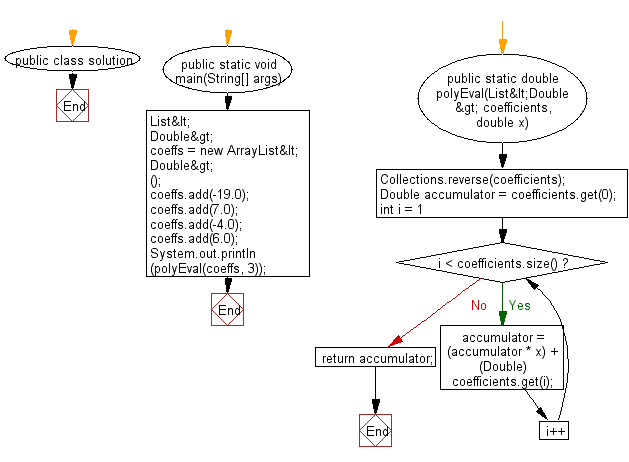﻿ Java Math Exercises: Compute the result from the innermost brackets - w3resource# Java Math Exercises: Compute the result from the innermost brackets

## Java Math Exercises: Exercise-23 with Solution

A fast scheme for evaluating a polynomial such as:
-19+ 7x- 4x2 + 6x3
when
x=3
is to arrange the computation as follows:((((0)x+6)x+(-4))x+7)x+(-19)
Write a Java program to compute the result from the innermost brackets.

Sample Solution:

Java Code:

``````import java.util.ArrayList;
import java.util.Collections;
import java.util.List;
public class solution {
public static void main(String[] args){
List<Double> coeffs = new ArrayList<Double>();
System.out.println(polyEval(coeffs, 3));
}
public static double polyEval(List<Double> coefficients, double x) {
Collections.reverse(coefficients);
Double accumulator = coefficients.get(0);
for (int i = 1; i < coefficients.size(); i++) {
accumulator = (accumulator * x) + (Double) coefficients.get(i);
}
return accumulator;
}
}
``````

Ref.: https://bit.ly/3chhDot

Sample Output:

```128.0
```

Flowchart:Java Code Editor:

What is the difficulty level of this exercise?

﻿

## Java: Tips of the Day

Parsing dates:

```import java.io.*;
import java.util.*;
import java.text.*;

String s = "2001/09/23 14:39";

SimpleDateFormat formatter = new SimpleDateFormat ("yyyy/MM/dd H:mm");
Date d = formatter.parse(s, new ParsePosition(0));
```